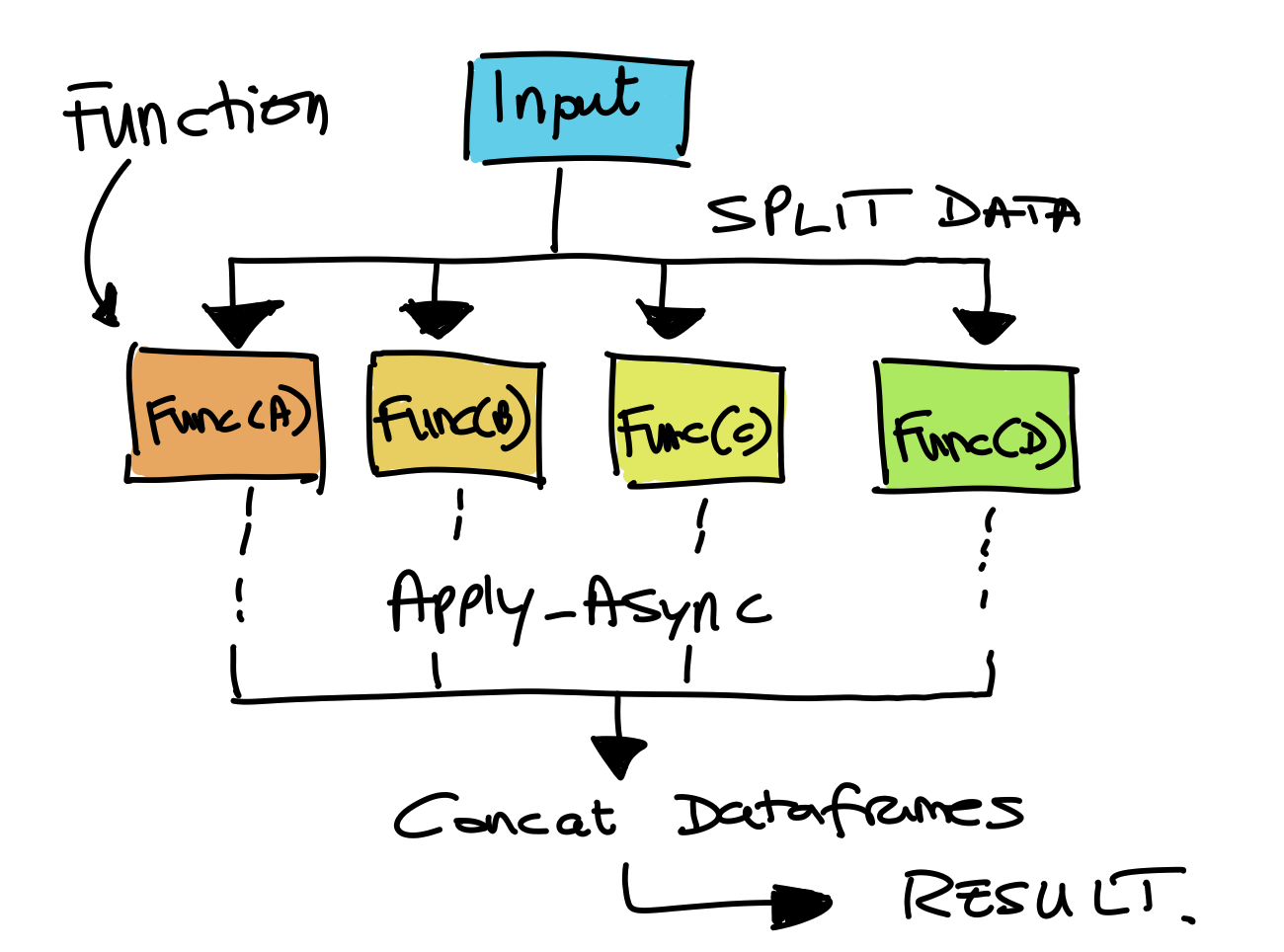# Parallelize a function in Python that returns a pandas DataFrame

April 10 2022

## Background

A common way to make functions run faster is to parallelize them. One way to achieve this in Python is to use the multiprocessing library. It can be tricky to get right though and won’t lend itself well to certain kinds of function. One application is when the function operates over a range of values that allow it to be split into smaller pieces, a subset of the whole. These pieces can then be joined together at the end. This is the case for the example shown here.Concept behind using this code.

## Bam file coverage

The function we want to speed up is one that gets the read coverage in a bam file. This is the result of an alignment of sequence reads against all the positions in a genome. The function uses subprocess to call samtools mpileup and gets the results into a dataframe. It can operate over a specific region of the genome specified by start and end positions. This means we can split the input into blocks or regions which can independently run and then combine the results at the end. multiprocessing works by creating a Pool object that tracks the processes. We call apply_async on this object supplying the function and arguments. (Here the single process version is _get_coverage). This returns an AsyncResult object and you call its get() method to retrieve the result of the function call. The list funclist is used to track the functions. We get the result from each one as they finish and add it to a list of dataframes. The functions can be run asynchronously here as the order we get the dataframes back in doesn’t matter per se as we can sort the final result later anyway.

Some readers may realise that samtools mpileup has been replaced by bcftools mpileup which it seems can be run multithreaded! So this method isn’t needed as such. However it is left here as a guide to applying the method in general.

## Code

from multiprocessing import  Pool
import pandas as pd
import numpy as np
import subprocess

def _get_coverage(bam_file, chr, start, end, ref):
"""
Get coverage of a region. Returns a dataframe.
"""

cmd = 'samtools mpileup {b} --min-MQ 10 -f {r} -r {c}:{s}-{e}'.format(c=chr,s=start,e=end,b=bam_file,r=ref)
temp = subprocess.check_output(cmd, shell=True)
if len(df)==0:
df['pos'] = range(start,end)
df['coverage']=0
return df

def get_coverage(bam_file, chr, start, end, ref, n_cores=8):
"""Get coverage from a bam file - parallelized.
Args:
bam_file: input bam file, should be indexed
chr: valid chromosome name
start/end: start and end positions for region
ref: reference genome used for alignment
"""

pool = Pool(n_cores)
x = np.linspace(start,end,n_cores,dtype=int)
blocks=[]
for i in range(len(x)):
if i < len(x)-1:
blocks.append((x[i],x[i+1]-1))

funclist = []
for start,end in blocks:
f = pool.apply_async(_get_coverage, [bam_file, chr, start, end, ref])
funclist.append(f)
result=[]
for f in funclist:
df = f.get(timeout=None)
result.append(df)
pool.close()
pool.join()
result = pd.concat(result)
#add zeros in missing positions - only needed because samtools ignore zero coverage positions
result = (result.set_index('pos')
.reindex(range(result.pos.iloc,result.pos.iloc[-1]+1), fill_value=0)
.reset_index())
return result


## Execution

A typical usage of this function is as follows:

df = rdiff.get_coverage('file.bam','NC_000962.3',1,4390000,mtb_genome,n_cores=12)


where mtb_genome is the name of a reference fasta file.

The output will look like this:

	pos	chr	base	coverage
0	4369000	NC_000962.3	T	204
1	4369001	NC_000962.3	G	205
2	4369002	NC_000962.3	C	222
3	4369003	NC_000962.3	T	219
4	4369004	NC_000962.3	C	220